Study Materials: ncert solutions

Our ncert solutions for Exercise 5.2 Class 10 maths 5. Arithmetic Progressions - ncert solutions - Toppers Study is the best material for English Medium students cbse board and other state boards students.

Solutions ⇒ Class 10th ⇒ Mathematics ⇒ 5. Arithmetic Progressions

# Exercise 5.2 Class 10 maths 5. Arithmetic Progressions - ncert solutions - Toppers Study

Topper Study classes prepares ncert solutions on practical base problems and comes out with the best result that helps the students and teachers as well as tutors and so many ecademic coaching classes that they need in practical life. Our ncert solutions for Exercise 5.2 Class 10 maths 5. Arithmetic Progressions - ncert solutions - Toppers Study is the best material for English Medium students cbse board and other state boards students.

## Exercise 5.2 Class 10 maths 5. Arithmetic Progressions - ncert solutions - Toppers Study

CBSE board students who preparing for class 10 ncert solutions maths and Mathematics solved exercise chapter 5. Arithmetic Progressions available and this helps in upcoming exams 2023-2024.

### You can Find Mathematics solution Class 10 Chapter 5. Arithmetic Progressions

• All Chapter review quick revision notes for chapter 5. Arithmetic Progressions Class 10
• NCERT Solutions And Textual questions Answers Class 10 Mathematics
• Extra NCERT Book questions Answers Class 10 Mathematics
• Importatnt key points with additional Assignment and questions bank solved.

NCERT Solutions do not only help you to cover your syllabus but also will give to textual support in exams 2023-2024 to complete exercise 5.2 maths class 10 chapter 5 in english medium. So revise and practice these all cbse study materials like class 10 maths chapter 5.2 in english ncert book. Also ensure to repractice all syllabus within time or before board exams for ncert class 10 maths ex 5.2 in english.

See all solutions for class 10 maths chapter 5 exercise 5 in english medium solved questions with answers.

### Exercise 5.2 class 10 Mathematics Chapter 5. Arithmetic Progressions

• Exercise 5.2 Class 10 Maths 5. Arithmetic Progressions - Ncert Solutions - Toppers Study
• Class 10 Ncert Solutions
• Solution Chapter 5. Arithmetic Progressions Class 10
• Solutions Class 10
• Chapter 5. Arithmetic Progressions Exercise 5.2 Class 10

## Exercise 5.2 Class 10 maths 5. Arithmetic Progressions - ncert solutions - Toppers Study

Exercise 5.2

Q1. Fill in the blanks in the following table, given that a is the first term, d the common difference and an the nth term of the AP:Q2. Choose the correct choice in the following and justify :
(i) 30th term of the AP: 10, 7, 4, . . . , is

(A) 97   (B) 77    (C) –77    (D) – 87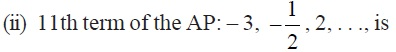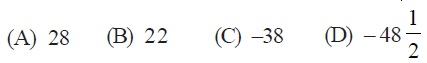Q3. In the following APs, find the missing terms in the boxes :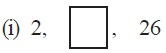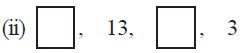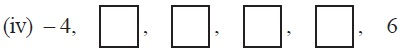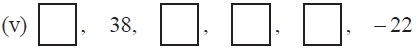Q4. Which term of the AP : 3, 8, 13, 18, . . . ,is 78?
Q5. Find the number of terms in each of the following APs :
(i) 7, 13, 19, . . . , 205Q6. Check whether – 150 is a term of the AP : 11, 8, 5, 2 . . .
Q7. Find the 31st term of an AP whose 11th term is 38 and the 16th term is 73.
Q8. An AP consists of 50 terms of which 3rd term is 12 and the last term is 106. Find the 29th term.
Q9. If the 3rd and the 9th terms of an AP are 4 and – 8 respectively, which term of this AP is zero?
Q10. The 17th term of an AP exceeds its 10th term by 7. Find the common difference.
Q11. Which term of the AP : 3, 15, 27, 39, . . . will be 132 more than its 54th term?
Q12. Two APs have the same common difference. The difference between their 100th terms is 100, what is the difference between their 1000th terms?
Q13. How many three-digit numbers are divisible by 7?
Q14. How many multiples of 4 lie between 10 and 250?
Q15. For what value of n, are the nth terms of two APs: 63, 65, 67, . . . and 3, 10, 17, . . . equal?
Q16. Determine the AP whose third term is 16 and the 7th term exceeds the 5th term by 12.

Q17. Find the 20th term from the last term of the AP : 3, 8, 13, . . ., 253.
Q18. The sum of the 4th and 8th terms of an AP is 24 and the sum of the 6th and 10th terms is 44. Find the first three terms of the AP.
Q19. Subba Rao started work in 1995 at an annual salary of Rs 5000 and received an increment of Rs 200 each year. In which year did his income reach Rs 7000?
Q20. Ramkali saved Rs 5 in the first week of a year and then increased her weekly savings by Rs 1.75. If in the nth week, her weekly savings become Rs 20.75, find n.

##### Other Pages of this Chapter: 5. Arithmetic Progressions

Important Study materials for classes 06, 07, 08,09,10, 11 and 12. Like CBSE Notes, Notes for Science, Notes for maths, Notes for Social Science, Notes for Accountancy, Notes for Economics, Notes for political Science, Noes for History, Notes For Bussiness Study, Physical Educations, Sample Papers, Test Papers, Mock Test Papers, Support Materials and Books.Mathematics Class - 11th

NCERT Maths book for CBSE Students.

books

## Study Materials List:

##### Solutions ⇒ Class 10th ⇒ Mathematics
1. Real Numbers
2. Polynomials
3. Pair of Linear Equations in Two Variables
5. Arithmetic Progressions
6. Triangles
7. Coordinate Geometry
8. Introduction to Trigonometry
9. Some Applications of Trigonometry
10. Circles
11. Constructions
12. Areas Related to Circles
13. Surface Areas and Volumes
14. Statistics
15. Probability

New Books